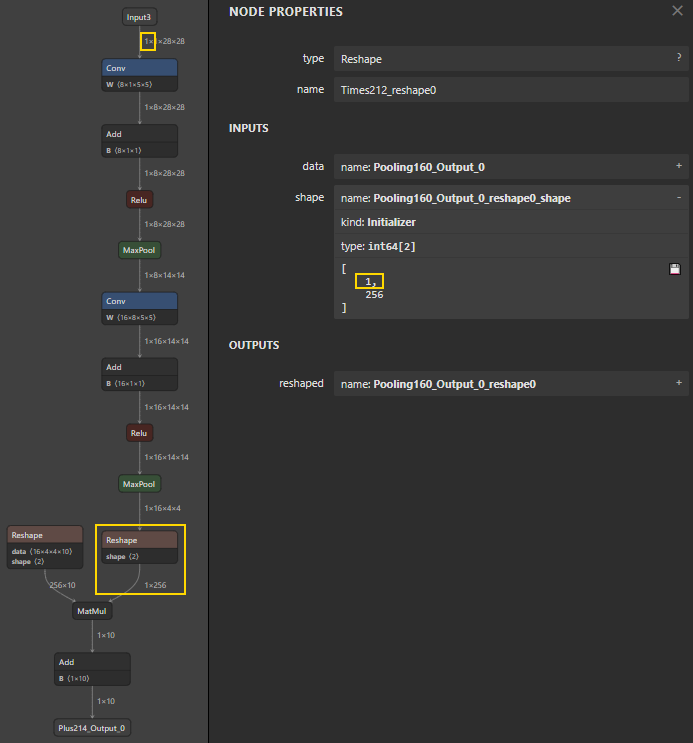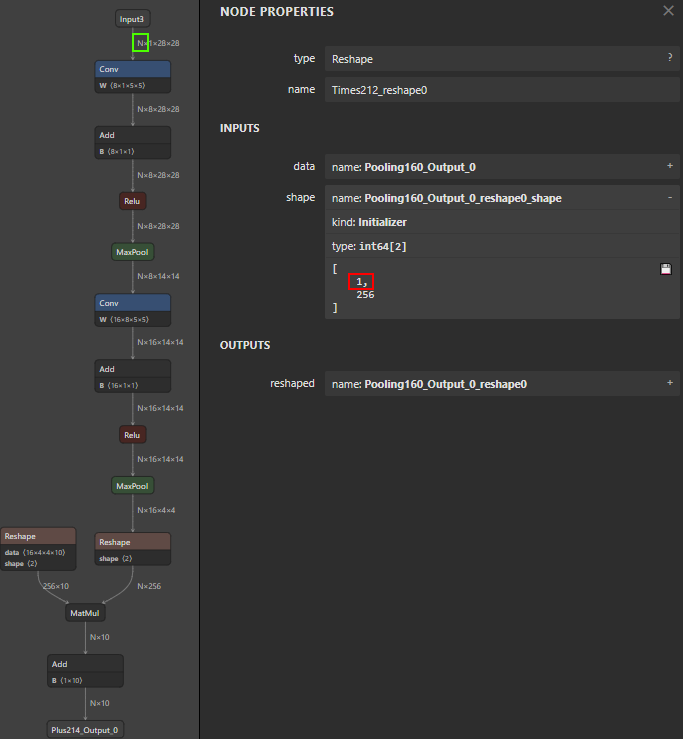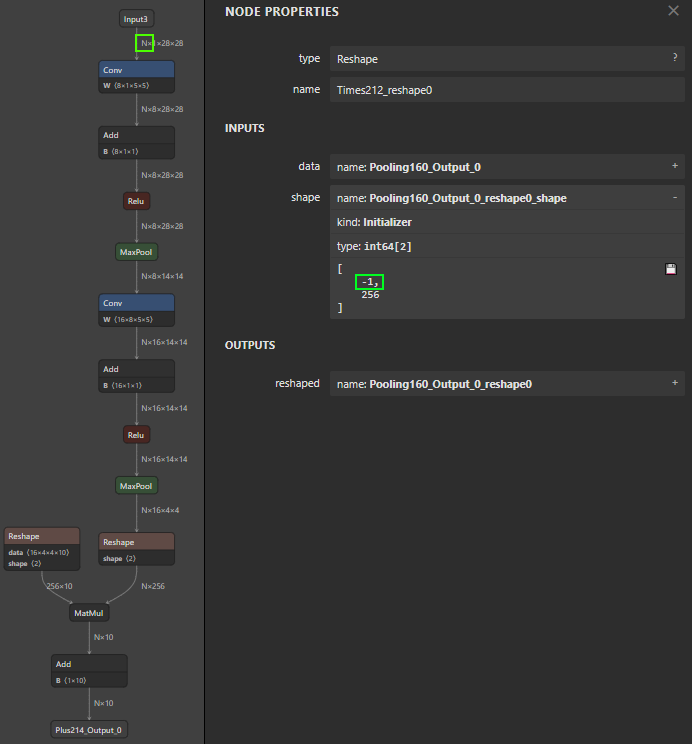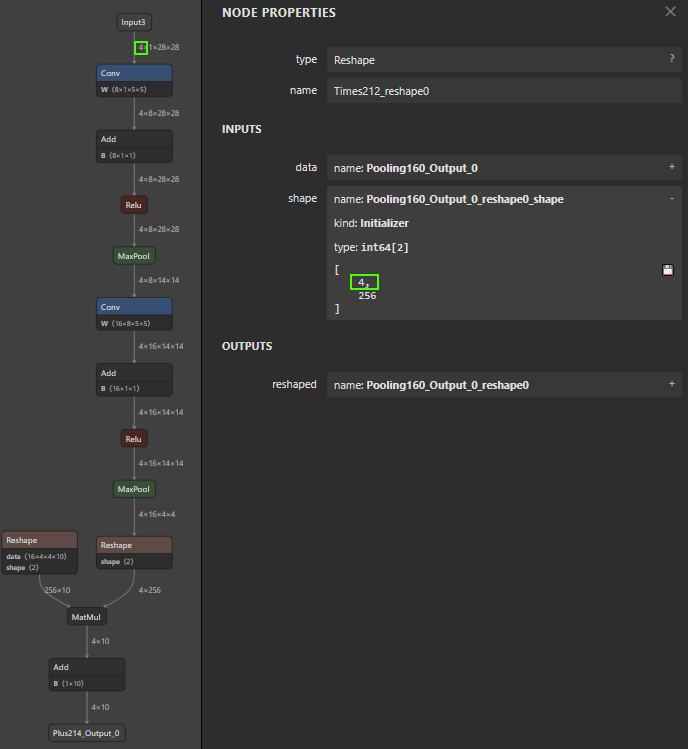# Set Dynamic Batch Size in ONNX Models using OnnxSharp

Continuing from Introducing OnnxSharp and ‘dotnet onnx’, in this post I will look at using OnnxSharp to set dynamic batch size in an ONNX model to allow the model to be used for batch inference using the ONNX Runtime:

• Setup: Inference using Microsoft.ML.OnnxRuntime
• Problem: Fixed Batch Size in Models
• Solution: OnnxSharp `SetDim`
• How: Don’t Forget Reshapes
• Notes: First Time Behavior

## Setup: Inference using Microsoft.ML.OnnxRuntime

To run inference using the ONNX Runtime I’ve create a small C# console project with the following files:

``````1
2
3
4
DynamicBatchSize.sln
DynamicBatchSize.csproj
Program.cs
mnist-8.onnx
``````

where `mnist-8-onnx` is the same model as discussed in the previous blog post.

The project file `DymamicBatchSize.csproj` contains:

``````1
2
3
4
5
6
7
8
9
10
11
12
13
14
15
16
17
18
19
20
<Project Sdk="Microsoft.NET.Sdk">

<PropertyGroup>
<OutputType>Exe</OutputType>
<TargetFramework>net5.0</TargetFramework>
<LangVersion>9.0</LangVersion>
</PropertyGroup>

<ItemGroup>
<PackageReference Include="Microsoft.ML.OnnxRuntime" Version="1.7.0" />
<PackageReference Include="OnnxSharp" Version="0.2.0" />
</ItemGroup>

<ItemGroup>
<None Update="mnist-8.onnx">
</None>
</ItemGroup>

</Project>
``````

and `Program.cs` in the new C# 9 top-level program style contains:

``````1
2
3
4
5
6
7
8
9
10
11
12
13
14
15
16
17
18
19
using System;
using System.Linq;
using Microsoft.ML.OnnxRuntime;
using Microsoft.ML.OnnxRuntime.Tensors;

using var inference = new InferenceSession("mnist-8.onnx");

NamedOnnxValue.CreateFromTensor(p.Key,
new DenseTensor<float>(p.Value.Dimensions)))
.ToArray<NamedOnnxValue>();

using var outputs = inference.Run(inputs);

var output = outputs.Single();
var outputTensor = output.AsTensor<float>();
var arrayString = outputTensor.GetArrayString();

Console.WriteLine(arrayString);
``````

This uses input meta-data to create a tensor for each input and corresponding `NamedOnnxValue` needed for ONNX Runtime inference. It is, therefore, easy to adapt to other models.

Running this outputs:

``````1
2
3
{
{-0.044856027,0.007791661,0.06810082,0.02999374,-0.12640963,0.14021875,-0.055284902,-0.049383815,0.08432205,-0.054540414}
}
``````

as you may have noticed this will be the output for a zero tensor of batch size 1 e.g. a black image. Recalling from the previous blog post the input is defined as:

Name Type ElemType Shape
Input3 TensorType Float 1x1x28x28

And output is defined as:

Name Type ElemType Shape
Plus214_Output_0 TensorType Float 1x10

That is, the input is a 28x28 image and output is a vector of 10 where largest output should correspond to predicted digit in the image.

## Problem: Fixed Batch Size in Models

To run with a different batch size we can change the code to the below. As can be seen we are simply changing the leading dimension of the input tensor from 1 to `batchSize`.

``````1
2
3
4
5
6
7
8
9
10
11
12
13
14
15
16
17
18
19
20
21
22
23
24
25
26
27
28
29
using System;
using System.Linq;
using Microsoft.ML.OnnxRuntime;
using Microsoft.ML.OnnxRuntime.Tensors;

using var inference = new InferenceSession("mnist-8.onnx");

const int batchSize = 2;

CreateNamedOnnxValueTensor(p.Key, p.Value, batchSize))
.ToArray<NamedOnnxValue>();

using var outputs = inference.Run(inputs);

var output = outputs.Single();
var outputTensor = output.AsTensor<float>();
var arrayString = outputTensor.GetArrayString();

Console.WriteLine(\$"N={batchSize} {arrayString}");

static NamedOnnxValue CreateNamedOnnxValueTensor(
string name, NodeMetadata node, int batchSize)
{
var dimensions = node.Dimensions;
dimensions = batchSize;
var tensor = new DenseTensor<float>(dimensions);
return NamedOnnxValue.CreateFromTensor(name, tensor);
}
``````

However, when running this it will throw an exception.

``````1
2
3
4
5
6
7
8
9
Unhandled exception. Microsoft.ML.OnnxRuntime.OnnxRuntimeException: [ErrorCode:InvalidArgument] Got invalid dimensions for input: Input3 for the following indices
index: 0 Got: 2 Expected: 1
Please fix either the inputs or the model.
at Microsoft.ML.OnnxRuntime.NativeApiStatus.VerifySuccess(IntPtr nativeStatus)
at Microsoft.ML.OnnxRuntime.InferenceSession.RunImpl(RunOptions options, IntPtr[] inputNames, IntPtr[] inputValues, IntPtr[] outputNames, DisposableList`1 cleanupList)
at <Program>\$.<Main>\$(String[] args) in Program.cs:line 15
``````

which has a clear message `index: 0 Got: 2 Expected: 1` indicating the model appears to be expecting the leading dimension or batch size to be 1.

This is a common problem with ONNX models, and can be an artifact of how ONNX models are exported from different ML frameworks. Most models are in fact defined with a dynamic batch size, since that is how they are trained, but when exporting to ONNX the exporter does not always handle this and instead simply outputs 1.

In the above the input tensor `Input3` shape is given as `1x1x28x28`. In this case this shape defines `NCHW` where:

• `N = 1` is the batch size
• `C = 1` is the number of channels e.g. gray (1) or RGB (3).
• `H = 28` is the height
• `W = 28` is the width

To fix this we need to change the `N` from `1` to, well, `"N"` in fact (that is any string) as that is how the ONNX format defines a dimension:

``````1
2
3
4
5
6
message Dimension {
oneof value {
int64 dim_value = 1;
string dim_param = 2;
};
};
``````

as can be seen in the ONNX protobuf schema onnx.proto3. That is, a dimension can be either a 64-bit signed integer or a string. When it is a string it is considered “dynamic” and the same string e.g. `"N"` can be used to indicate the same dimension flowing through the graph from inputs to outputs.

So how can we change this?

## Solution: OnnxSharp`SetDim`

With OnnxSharp it is very easy to change the fixed leading dimension using `SetDim` as shown below:

``````1
2
3
4
5
6
7
8
9
10
11
12
13
14
15
16
17
18
19
20
21
22
23
24
25
26
27
28
29
30
31
32
33
34
35
using System;
using System.Linq;
using Microsoft.ML.OnnxRuntime;
using Microsoft.ML.OnnxRuntime.Tensors;
using Onnx;

var model = ModelProto.Parser.ParseFromFile("mnist-8.onnx");
model.Graph.SetDim();
var modelBytes = model.ToByteArray();

using var inference = new InferenceSession(modelBytes);

const int batchSize = 2;

CreateNamedOnnxValueTensor(p.Key, p.Value, batchSize))
.ToArray<NamedOnnxValue>();

using var outputs = inference.Run(inputs);

var output = outputs.Single();
var outputTensor = output.AsTensor<float>();
var arrayString = outputTensor.GetArrayString();

Console.WriteLine(\$"N={batchSize} {arrayString}");

static NamedOnnxValue CreateNamedOnnxValueTensor(
string name, NodeMetadata node, int batchSize)
{
var dimensions = node.Dimensions;
dimensions = batchSize;
var tensor = new DenseTensor<float>(dimensions);
return NamedOnnxValue.CreateFromTensor(name, tensor);
}
``````

which simply loads the ONNX model first via `OnnxSharp` calls `SetDim()` on the graph which defaults to changing the leading dimension to `"N"`. This also has an overload allowing for customization e.g.:

``````1
2
public static void SetDim(this GraphProto graph,
int dimIndex, DimParamOrValue dimParamOrValue);
``````

That is, `SetDim()` is equivalent to calling:

``````1
model.Graph.SetDim(dimIndex: 0, DimParamOrValue.New("N"));
``````

After changing the dimension the model can either be converted to a byte array or saved to file. In this case, I’m simply converting to a byte array which we can forward directly to the `InferenceSession` constructor.

You can also use the `dotnet onnx` tool to do the same if you prefer that with:

``````1
dotnet onnx setdim mnist-8.onnx mnist-8-setdim.onnx
``````

Running this code will then output:

``````1
2
3
4
N=2 {
{-0.044856027,0.007791661,0.06810082,0.02999374,-0.12640963,0.14021875,-0.055284902,-0.049383815,0.08432205,-0.054540414},
{-0.044856027,0.007791661,0.06810082,0.02999374,-0.12640963,0.14021875,-0.055284902,-0.049383815,0.08432205,-0.054540414}
}
``````

Success! We can also change the batch size to `8`:

``````1
2
3
4
5
6
7
8
9
10
N=8 {
{-0.044856027,0.007791661,0.06810082,0.02999374,-0.12640963,0.14021875,-0.055284902,-0.049383815,0.08432205,-0.054540414},
{-0.044856027,0.007791661,0.06810082,0.02999374,-0.12640963,0.14021875,-0.055284902,-0.049383815,0.08432205,-0.054540414},
{-0.044856027,0.007791661,0.06810082,0.02999374,-0.12640963,0.14021875,-0.055284902,-0.049383815,0.08432205,-0.054540414},
{-0.044856027,0.007791661,0.06810082,0.02999374,-0.12640963,0.14021875,-0.055284902,-0.049383815,0.08432205,-0.054540414},
{-0.044856027,0.007791661,0.06810082,0.02999374,-0.12640963,0.14021875,-0.055284902,-0.049383815,0.08432205,-0.054540414},
{-0.044856027,0.007791661,0.06810082,0.02999374,-0.12640963,0.14021875,-0.055284902,-0.049383815,0.08432205,-0.054540414},
{-0.044856027,0.007791661,0.06810082,0.02999374,-0.12640963,0.14021875,-0.055284902,-0.049383815,0.08432205,-0.054540414},
{-0.044856027,0.007791661,0.06810082,0.02999374,-0.12640963,0.14021875,-0.055284902,-0.049383815,0.08432205,-0.054540414}
}
``````

## How: Don’t Forget Reshapes

From the above it may seem straightforward to change a model from fixed batch size of `1` to `N` by simply replacing the leading dimension in all inputs (except initializer inputs), value infos and outputs in the graph. This is also what appears to be the most common cited solution on the web with accompanying Python code. However, as always the devil is in the details.

I mostly work with CNN models. Most of these are based on a CNN back-end and some form of dense front-end. In the transition from “3D” (CHW) back-end to “1D” front-end there often is a reshape to handle this. This can be seen below for the `mnist-8.onnx` model, with the right side `Reshape` node called `"Times212_reshape0"`.

Reshape nodes have they operation specified by an accompanying “shape” tensor that defines the dimensions of the reshape. In this case it is `int64 = [ 1, 256 ]`. The reshape is, therefore, fixed to this shape. This is again an artefact of the ONNX exporter not handling dynamic shapes and instead outputting fixed size leading dimensions.If this is not handled when changing the leading dimension, the ONNX Runtime will fail with an exception:

``````1
2
3
4
5
6
7
8
9
10
11
12
13
14
15
16
17
18
[E:onnxruntime:, sequential_executor.cc:339 onnxruntime::SequentialExecutor::Execute]
Non-zero status code returned while running Reshape node.
Name:'Times212_reshape0' Status Message:
D:\a\_work\1\s\onnxruntime\core\providers\cpu\tensor\reshape_helper.h:43 onnxruntime::ReshapeHelper::ReshapeHelper gsl::narrow_cast<int64_t>(input_shape.Size()) == size was false.
The input tensor cannot be reshaped to the requested shape.
Input shape:{2,16,4,4}, requested shape:{1,256}

Unhandled exception. Microsoft.ML.OnnxRuntime.OnnxRuntimeException:
[ErrorCode:RuntimeException] Non-zero status code returned while running Reshape node.
Name:'Times212_reshape0' Status Message: D:\a\_work\1\s\onnxruntime\core\providers\cpu\tensor\reshape_helper.h:43 onnxruntime::ReshapeHelper::ReshapeHelper gsl::narrow_cast<int64_t>(input_shape.Size()) == size was false.
The input tensor cannot be reshaped to the requested shape. Input shape:{2,16,4,4}, requested shape:{1,256}

at Microsoft.ML.OnnxRuntime.NativeApiStatus.VerifySuccess(IntPtr nativeStatus)
at Microsoft.ML.OnnxRuntime.InferenceSession.RunImpl(RunOptions options, IntPtr[] inputNames, IntPtr[] inputValues, IntPtr[] outputNames, DisposableList`1 cleanupList)
at <Program>\$.<Main>\$(String[] args) in Program.cs:line 20
``````

Which can be hard to understand unless you check the reshape operation in detail as shown below. Where all the leading dimensions have been changed to `N` but the reshape shape has not been changed. Note that the graph contains another reshape, that is not changing, this is because this is for an initializer input. OnnxSharp handles all this. For details on how `SetDim` works see source, which at this time still needs a bit of cleanup.Using OnnxSharp to set dynamic batch size will instead make sure the reshape is changed to being dynamic by changing the given dimension to `-1` which is what the Reshape operation uses to define a dynamic dimension. Only 1 of the dimensions in the shape can be -1, though. This can be seen below.It is also possible to use `SetDim` to simply set a new fixed batch size as shown below. This can be useful when taking into account how the ONNX Runtime works as discussed next.

``````1
model.Graph.SetDim(dimIndex: 0, DimParamOrValue.New(4));
``````## Notes: First Time Behavior

When running a model with only fixed dimensions the ONNX Runtime will (depending on execution provider) prepare and optimize the graph for execution when constructing the `InferenseSession` that is in the call:

``````1
using var inference = new InferenceSession("mnist-8.onnx");
``````

For large models and e.g. using the TensorRT execution provider this means this call can take minutes (depending on model of course) to execute if doing full graph optimizations.

However, when the model has dynamic dimensions like batch size this is not the case. Instead, the ONNX Runtime may instead cache optimized graphs (again it depends on execution provider, some don’t) for specific batch sizes when inputs are first encountered for that batch size. This means the first time `Run` is called is when the graph is optimized:

``````1
using var outputs = inference.Run(inputs);
``````

Meaning this `Run` call can now take minutes to complete for a new batch size. Depending on use case it is, therefore, a good idea to call `Run` at least once for each expected batch size upon startup to avoid this in the middle of production.

Or if only one batch size is used during production, set a fixed batch size using `SetDim` as discussed above. In any case `OnnxSharp` can help. 😀

I don’t expect `OnnxSharp` to handle all cases relevant for this, some of the code assumes a dimension to be 1 before being replaced for example. If you encounter an ONNX model that doesn’t work, then feel free to open an issue on GitHub.

2021.05.24